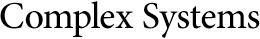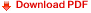## Some Relativistic and Gravitational Properties of the Wolfram ModelJonathan Gorard
University of Cambridge
jg865@cam.ac.uk
and
Wolfram Research, Inc.
jonathang@wolfram.com

#### Abstract

The Wolfram Model, which is a slight generalization of the model first introduced by Stephen Wolfram in A New Kind of Science (NKS) , is a discrete spacetime formalism in which space is represented by a hypergraph whose dynamics are determined by abstract replacement operations on set systems, and in which the conformal structure of spacetime is represented by a causal graph. The purpose of this paper is to present rigorous mathematical derivations of many key properties of such models in the continuum limit, as first discussed in NKS, including the fact that large classes of them obey discrete forms of both special and general relativity. First, we prove that causal invariance (namely, the requirement that all causal graphs be isomorphic, irrespective of the choice of hypergraph updating order) is equivalent to a discrete version of general covariance, with changes to the updating order corresponding to discrete gauge transformations. This fact then allows one to deduce a discrete analog of Lorentz covariance, and the resultant physical consequences of discrete Lorentz transformations. We also introduce discrete notions of Riemann and Ricci curvature for hypergraphs, and prove that the correction factor for the volume of a discrete spacetime cone in a causal graph corresponding to curved spacetime of fixed dimensionality is proportional to a timelike projection of the discrete spacetime Ricci tensor, subsequently using this fact (along with the assumption that the updating rules preserve the dimensionality of the causal graph in limiting cases) to prove that the most general set of constraints on the discrete spacetime Ricci tensor corresponds to a discrete form of the Einstein field equations. Finally, we discuss a potential formalism for general relativity in hypergraphs of varying local dimensionality, and the implications that this formalism may have for inflationary cosmology and the value of the cosmological constant. Connections to many other fields of mathematics—including mathematical logic, abstract rewriting systems, λ-calculus, category theory, automated theorem-proving, measure theory, fluid mechanics, algebraic topology and geometric group theory—are also discussed.

Cite this publication as:
J. Gorard, “Some Relativistic and Gravitational Properties of the Wolfram Model,” Complex Systems, 29(2), 2020 pp. 599–654.
https://doi.org/10.25088/ComplexSystems.29.2.599